The Star Polygons ClipArt gallery includes 33 images of star-shaped polygons. A polygon is a closed geometric figure with sides made up of line segments. Star-shaped polygons are concave polygons that resemble a star.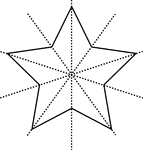### Star, 5-Point

Star with 5 points with lines of symmetry.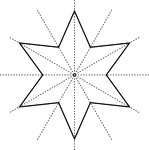### Star, 6-Point

Star with 6 points with lines of symmetry.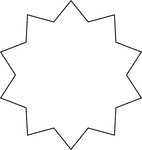### 10-Point Star

Illustration of a closed concave geometric figure with 20 sides in the shape of a 10-point star.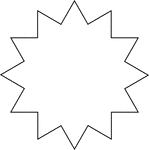### 12-Point Star

Illustration of a closed concave geometric figure with 24 sides in the shape of a 12-point star.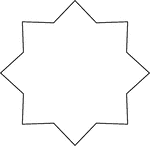### 8-Point Star

Illustration of a closed concave geometric figure with 16 sides in the shape of a 8-point star.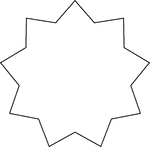### 9-Point Star

Illustration of a closed concave geometric figure with 18 sides in the shape of a 9-point star.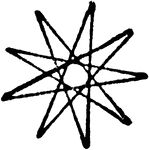### Triangular Nine Point Nanogram Star

A triangular nanogram star with 9 points. This nine point star is created by drawing nine diagonal lines…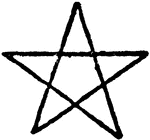### Pentagram Star

A pentagram star. The five point star is drawn by four straight diagonal lines. The pentagram star was…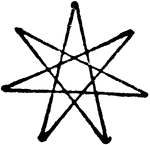### Seven Point Heptagram Star

A heptagram star with seven points. The star is drawn by 7 diagonal lines.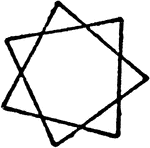### Six Point Squared Heptagram Star

The illustration of a square heptagram star with six points. A heptagon polygon can make two stars.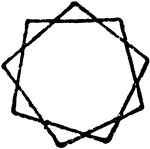### Squared Eight Point Nanogram Star

A squared nanogram star with 8 points. The star is created by using two four sided squares on top of…